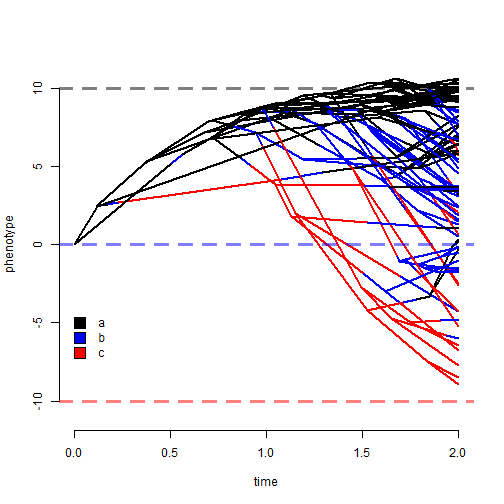## Sunday, March 12, 2017

### Difference equation approximation OU simulation function

For reasons I won't get into in detail, I just wrote a simple function to conduct Ornstein-Uhlenbeck simulations by finely discretizing time and then using a simple difference equation version of the OU model to simulate as follows:

``````multiOU<-function(tree,alpha,sig2,theta=NULL,a0=NULL,internal=TRUE,...){
if(hasArg(dt)) dt<-list(...)\$dt
else dt<-1/1000*max(nodeHeights(tree))
ss<-sort(unique(c(getStates(tree,"tips"),getStates(tree,"nodes"))))
if(is.null(theta)) theta<-setNames(rep(0,length(ss)),ss)
if(is.null(a0)) a0<-0
root<-Ntip(tree)+1
S<-matrix(NA,nrow(tree\$edge),2)
S[which(tree\$edge[,1]==root),1]<-a0
for(i in 1:nrow(tree\$edge)){
x1<-S[i,1]
for(j in 1:length(tree\$maps[[i]])){
t<-tree\$maps[[i]][j]
ALPHA<-alpha[names(t)]
SIG2<-sig2[names(t)]
THETA<-theta[names(t)]
t<-c(rep(dt,floor(t/dt)),t%%dt)
for(k in 1:length(t))
x1<-x1+ALPHA*(THETA-x1)*t[k]+
rnorm(n=1,sd=sqrt(SIG2*t[k]))
}
S[i,2]<-x1
if(any(tree\$edge[,1]==tree\$edge[i,2]))
S[which(tree\$edge[,1]==tree\$edge[i,2]),1]<-S[i,2]
}
x<-setNames(c(S[1,1],S[,2]),c(tree\$edge[1,1],
tree\$edge[,2]))[as.character(1:(Ntip(tree)+tree\$Nnode))]
names(x)[1:Ntip(tree)]<-tree\$tip.label
if(!internal) x<-x[tree\$tip.label]
x
}
``````

In the following, I'll use it to simulate:

``````library(phytools)
set.seed(116)
cols<-setNames(c("black","blue","red"),letters[1:3])
tree<-pbtree(n=100,scale=2)
Q<-matrix(c(-1,1,0,1,-2,1,0,1,-1),3,3,
dimnames=list(letters[1:3],letters[1:3]))
tree<-sim.history(tree,Q,anc="a")
``````
``````## Done simulation(s).
``````
``````sig2<-setNames(c(1,2,1),letters[1:3])
alpha<-setNames(c(2,2,2),letters[1:3])
theta<-setNames(c(10,0,-10),letters[1:3])
x<-multiOU(tree,alpha,sig2,theta)
phenogram(tree,x,ftype="off",colors=cols,ylim=c(-11,11),
xlim=c(0,2))
abline(h=c(-10,0,10),lty="dashed",col=sapply(c("red","blue",
"black"),make.transparent,alpha=0.5),lwd=3,lend=2)
``````I'm going to add (importantly) that the same simulation is done without the discrete difference equation approximation by `OUwie.sim` in the powerful OUwie package by Jeremy Beaulieu & Brian O'Meara.

Finally, let's fit the appropriate 'OUwie' (that is, flexible OU) model to these data:

``````library(OUwie)
data<-data.frame(Genus_species=tree\$tip.label,
Reg=getStates(tree,"tips"),X=as.numeric(x[tree\$tip.label]))
OUwie(tree,data,model="OUMV",simmap.tree=TRUE)
``````
``````## Initializing...
## Finished. Begin thorough search...
## Finished. Summarizing results.
``````
``````##
## Fit
##       lnL      AIC     AICc model ntax
##  -153.169 320.3379 321.5553  OUMV  100
##
##
## Rates
##                  a         b         c
## alpha    1.6410055  1.641006  1.641006
## sigma.sq 0.8331012 12.695801 73.799702
##
## Optima
##                  a          b         c
## estimate 9.5391941 -2.5029839 -14.22271
## se       0.1269122  0.8906037   2.51031
##
## Arrived at a reliable solution
``````

One of our σ2 estimates is way off - but aside from that, we seem to have more or less captured the generating process. Cool.